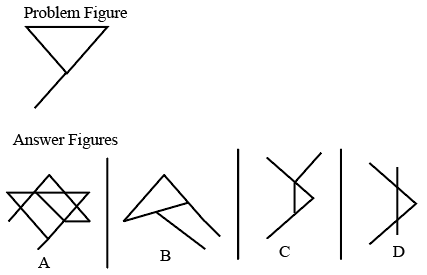Question 1

# The given Problem Figure is embedded in one of the given Answer Figures. Which is that Answer Figure?Solution

If you see the given figure ,

it is taken from the figure A in the above options.

we can get it from following way,

So, option D is correct choice.